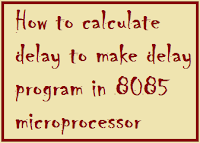## Sunday, 8 September 2013

### How to calculate delay to make delay program in 8085 microprocessorWe discus on my earlier post that each instruction passes through different combinations of Fetch, Memory Read, and Memory Write cycles at http://amienbcafe.blogspot.in/2013/08/video-tutorial-on-how-to-draw-timing.html.
Now if you know the combinations of cycles then you can calculate how long such an instruction would require to complete.
To know how many T-States an instruction requires, and keeping in mind
that a T-State is one clock cycle long, we can calculate the time using the following formula:

Delay = No. of T-States / Frequency

Now let we take a example to understand easily.
If the speed of your microprocessor is 2 MHz then for a “MVI” instruction uses 7 T-States. Therefore the instruction would require 3.5 μ Seconds to complete.

Now we can use a loop to produce a certain amount of time delay in a program.

See an example of a delay loop:

MVI C, FFH                              7 T-States
LOOP:     DCR C                                     4 T-States
JNZ LOOP                              10 T-States

Here see the first instruction initializes the loop counter and is executed only once requiring only 7 T-States.

The following two instructions which is inside a loop that requires 14 T-States to execute and is repeated 255 times until C becomes 0.

We need to keep in mind though that in the last rotation of the loop, the JNZ instruction will not jump to the address and so it requires only 7 T-States rather than the 10.

•Therefore, we must deduct 3 T-States from the total delay to get an accurate delay calculation.
•To calculate the delay, we use the following formula:

Tdelay =  TO + TL

Tdelay= total delay
TO= delay outside the loop
TL= delay of the loop

Now using these formulas, we can calculate the time delay for the above mansion example:

•TO= 7 T-States Delay of the MVI instruction

•TL= (14 X 255) -3 = 3567 T-States (14 T-States for the 2 instructions repeated 255 times).

Now your microprocessor speed is 2 MHz. so we know
T (time for one T state) = 1/ F
= ½  X 10-6 sec
= .5 µ sec

So now we have 3567 T-state so we will get 3567 X .5 µ sec = 1.7835 m sec of delay.

1.2.3.4.